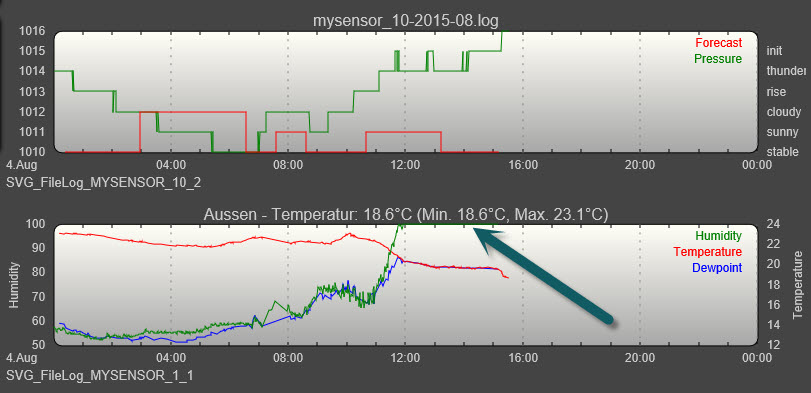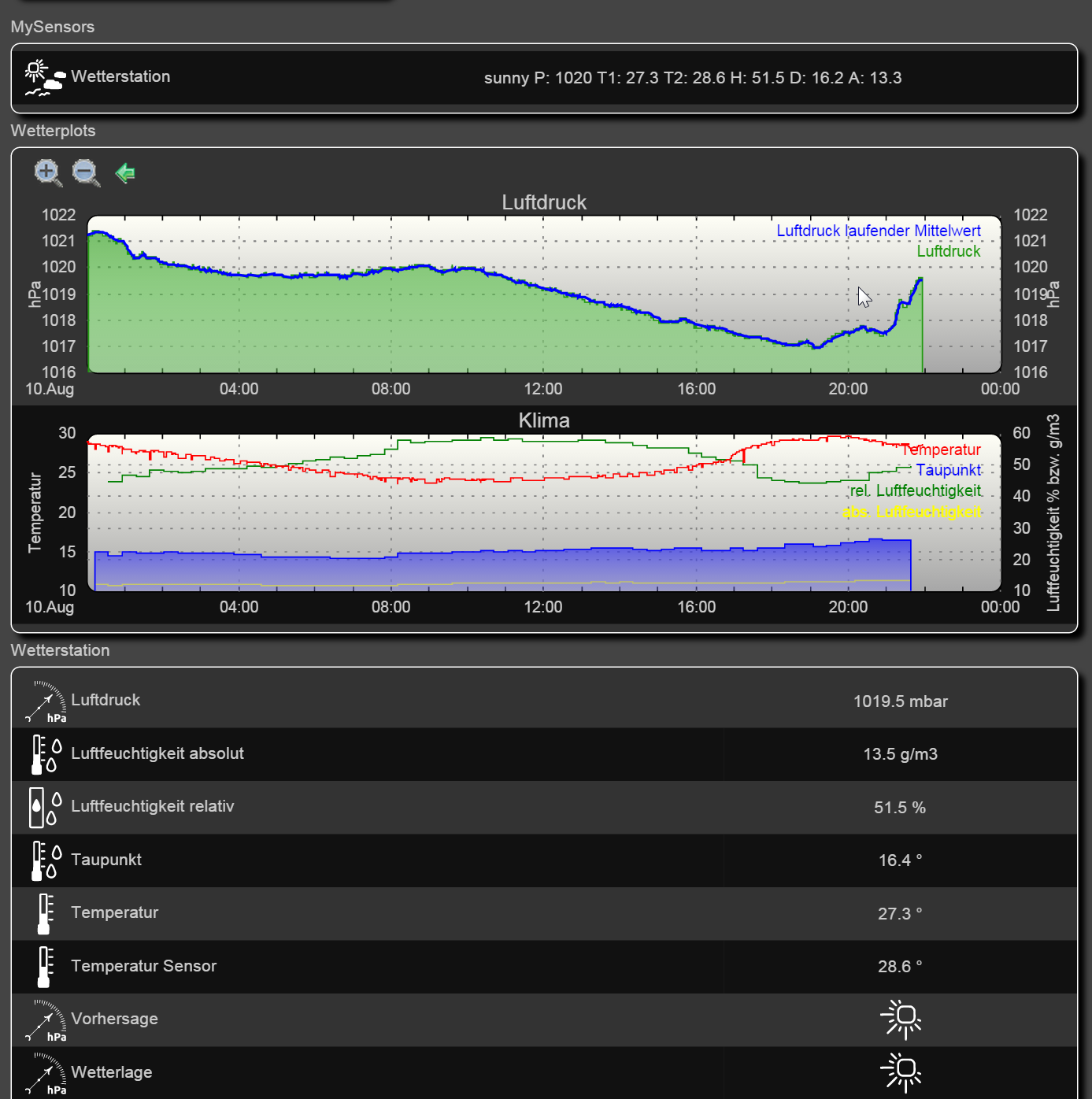# Pressure example BMP085

• I had a closer look at the code for the pressure example (http://www.mysensors.org/build/pressure) and was a little bit
confused about the weather forecast algorithm. It should follow the algorithm proposed here:
http://www.freescale.com/files/sensors/doc/app_note/AN3914.pdf

The sketch takes the pressure every minute and stores the value in a global array. The array has room for 180 sample which makes 3 hours.
Every 30 minutes the average of the last 5 minutes is taken and compared to the average values in the past to find if the pressure is raising or not. Well that seems to be an easy job, but the code looks really ugly and consumes too much memory.

First of all I would only store the last 5 samples instead of all 180, as the other samples are not used anyway in the whole sketch.
Then I think there is an error when minute 180 is reached. The sketch stores the average5 into average0 (Line 169) and sets the minute counter to 5 (Line 99) which immediately triggers the calculation of average0 agaein (line 105) which is based on old data (minutes 0-4).
I think the minute counter should be set to 6 here.

I reached the memory limit of my arduino nano when merging the code for several sensors together. So I had a deeper look at the code and changed it like below:

globals:

``````const char *weather[] = { "stable", "sunny", "cloudy", "unstable", "thunderstorm", "unknown" };
enum FORECAST
{
STABLE = 0,			// Stable weather
SUNNY = 1,			// Slowly rising HP stable good weather
CLOUDY = 2,			// Slowly falling Low Pressure System, stable rainy weather
UNSTABLE = 3,		// Quickly rising HP, not stable weather
THUNDERSTORM = 4,	// Quickly falling LP, Thunderstorm, not stable
UNKNOWN = 5			// Unknown, more time needed
};

const int LAST_SAMPLES_COUNT = 5;
float lastPressureSamples[LAST_SAMPLES_COUNT];
#define MULTIPLIER (65.0/1023.0)

int minuteCount = 0;
bool firstRound = true;
float pressureAvg;
float dP_dt;
``````

algorithm

``````float getLastPressureSamplesAverage()
{
float lastPressureSamplesAverage = 0;
for (int i = 0; i < LAST_SAMPLES_COUNT; i++)
{
lastPressureSamplesAverage += lastPressureSamples[i];
}
lastPressureSamplesAverage /= LAST_SAMPLES_COUNT;

return lastPressureSamplesAverage ;
}

// Algorithm found here
// http://www.freescale.com/files/sensors/doc/app_note/AN3914.pdf
int sample(float pressure) {

// Calculate the average of the last n minutes.
int index = minuteCount % LAST_SAMPLES_COUNT;
lastPressureSamples[index] = pressure;

minuteCount++;
if (minuteCount > 185)
minuteCount = 6;

if (minuteCount == 5) {
pressureAvg = getLastPressureSamplesAverage();
}
else if (minuteCount == 35) {
pressureAvg = getLastPressureSamplesAverage();
float change = (pressureAvg - pressureAvg);
if (firstRound) // first time initial 3 hour
dP_dt = (MULTIPLIER * 2 * change); // note this is for t = 0.5hour
else
dP_dt = ((MULTIPLIER * change) / 1.5); // divide by 1.5 as this is the difference in time from 0 value.
}
else if (minuteCount == 65) {
pressureAvg = getLastPressureSamplesAverage();
float change = (pressureAvg - pressureAvg);
if (firstRound) //first time initial 3 hour
dP_dt = (MULTIPLIER * change); //note this is for t = 1 hour
else
dP_dt = ((MULTIPLIER * change) / 2); //divide by 2 as this is the difference in time from 0 value
}
else if (minuteCount == 95) {
pressureAvg = getLastPressureSamplesAverage();
float change = (pressureAvg - pressureAvg);
if (firstRound) // first time initial 3 hour
dP_dt = ((MULTIPLIER * change) / 1.5); // note this is for t = 1.5 hour
else
dP_dt = ((MULTIPLIER * change) / 2.5); // divide by 2.5 as this is the difference in time from 0 value
}
else if (minuteCount == 125) {
pressureAvg = getLastPressureSamplesAverage();
float change = (pressureAvg - pressureAvg);
if (firstRound) // first time initial 3 hour
dP_dt = ((MULTIPLIER * change) / 2); // note this is for t = 2 hour
else
dP_dt = ((MULTIPLIER * change) / 3); // divide by 3 as this is the difference in time from 0 value
}
else if (minuteCount == 155) {
pressureAvg = getLastPressureSamplesAverage();
float change = (pressureAvg - pressureAvg);
if (firstRound) // first time initial 3 hour
dP_dt = ((MULTIPLIER * change) / 2.5); // note this is for t = 2.5 hour
else
dP_dt = ((MULTIPLIER * change) / 3.5); // divide by 3.5 as this is the difference in time from 0 value
}
else if (minuteCount == 185) {
pressureAvg = getLastPressureSamplesAverage();
float change = (pressureAvg - pressureAvg);
if (firstRound) // first time initial 3 hour
dP_dt = ((MULTIPLIER * change) / 3); // note this is for t = 3 hour
else
dP_dt = ((MULTIPLIER * change) / 4); // divide by 4 as this is the difference in time from 0 value

pressureAvg = pressureAvg; // Equating the pressure at 0 to the pressure at 2 hour after 3 hours have past.
firstRound = false; // flag to let you know that this is on the past 3 hour mark. Initialized to 0 outside main loop.
}

int forecast = UNKNOWN;
if (minuteCount < 35 && firstRound) //if time is less than 35 min on the first 3 hour interval.
forecast = UNKNOWN;
else if (dP_dt < (-0.25))
forecast = THUNDERSTORM;
else if (dP_dt > 0.25)
forecast = UNSTABLE;
else if ((dP_dt > (-0.25)) && (dP_dt < (-0.05)))
forecast = CLOUDY;
else if ((dP_dt > 0.05) && (dP_dt < 0.25))
forecast = SUNNY;
else if ((dP_dt >(-0.05)) && (dP_dt < 0.05))
forecast = STABLE;
else
forecast = UNKNOWN; // Unknown

return forecast;
``````

}

Could anyone verify this as I could easily be wrong.

• According to Samuel the calculation of the forecast is wrong:
He wrote:

The forecast algorithm is incorrect. In the code you do dP_dt = (((65.0 / 1023.0) * change) / 3). However, there is no reason to multiply the change by 65/1023. In the source, they do this because their barometer is like that : when their barometer return 1, it means 1 * 65.0 / 1023.0 kPa. In our sketch, when our barometer return 1, it means 1 Pa, and in this part of the code pressure is in hPa so we should divide by 10.0 Should be in kPa/h.

I guess someone should rework the whole sketch.

• So (and not to cause issue), is the sketch as it stands returning an incorrect value, and just inefficient? Or is the value being returned incorrect as well?

I'm definitely all for streamlining the code, especially if it is storing arrays of a lot of unused figures. I use a BMP085 around here and in testing, and it is in a location where I want to have a few sensors on the one unit, as it will essentially form my main weather station, so the reworking of the code would be interesting.

I'm not too up to scratch on the code side, still only just learning the semantics of arduino, so I would be keen to see how this one develops. Nice work!• The forecast of my weatherstation is always "stable". The wrong factor could be an explanation for that. I changed the sketch some minutes ago, now I am curious if the forcast algorithm works as expected.

• @Heinz Yesterday we had a thunder forecast... but the sensor reported cloudy so i will also try thisThank you! I will try and report back - well done!

• Well, after the changes the forecast obviously works well. I will try to submit the changed code the next days.

• For all the v2 library is out, although the v1 is the one commonly provided today

The BMP085 has been discontinued by BOSCH and replaced with the BMP180 which is smaller and less expensive but is identical in terms of wiring and software!

to have a correct pressure you need to correct this:

`````` float pressure_raw = bmp.readPressure();
+  float pressure = pressure_raw/pow((1.0 - ( myAltitude/44330.0 )), 5.255);
``````

now I have values that I can validate with other barometers. You just need to know your altitude from sea level.

• The library used in the pressure example of the mysensors project already supports this:

``````	float pressure = bmp.readSealevelPressure(ALTITUDE) / 100.0;
``````

Btw I fixed some issues in the sample see also
https://github.com/mysensors/Arduino/tree/master/libraries/MySensors/examples/PressureSensor

• Hi all,

the forcast is working as expected for a while now. It follows the pressure values from the device.

First diagram shows pressure and forcast. Looks like the calculation is ok.
But the weather doesnt know anything about the forecast100% Humidity because it rains Dogs and CatsThis is no question. Just a little reminder about crystalballsAndreas

• Mine is also working well...here is my FHEM screentshot of today. Right now it is clearing up again after a short thunder storm...I also added a weather situation indicator which works like a common barometer: it shows sunny when the pressure is above 1013hPa and rainy if the pressure is below this world wide average.

The field "Vorhersage" shows the forecast "sunny" as the pressure raised last last hours significantly.

• @Heinz
I too am having reading issues with the new 180 pressure sensor.

a couple of questions,
Is altitude stated in meters I think?

The code revisions;
Are they to added to sketch in place of the existing parts, while the algorithm is to be totally replaced with the revised code you have supplied?

Thanks

• @5546dug
Yes, the altitude is in meters.
A complete sketch containing the changes and some bug fixes is part of the mysensors library 1.5. So you don't have to merge the code from above, simply download it from github.

• @Heinz thanks I am still on 1.4.1 and will look it up

• @Heinz
Well once again I am stumped, I can't find this library I need, do I need to upgrade my system to 1.5 to find this file., or am I just not seeing what I need to see?• You don't need to upgrade to 1.5, you can simply download the sketch from here
https://github.com/mysensors/Arduino/tree/development/libraries/MySensors/examples/PressureSensor